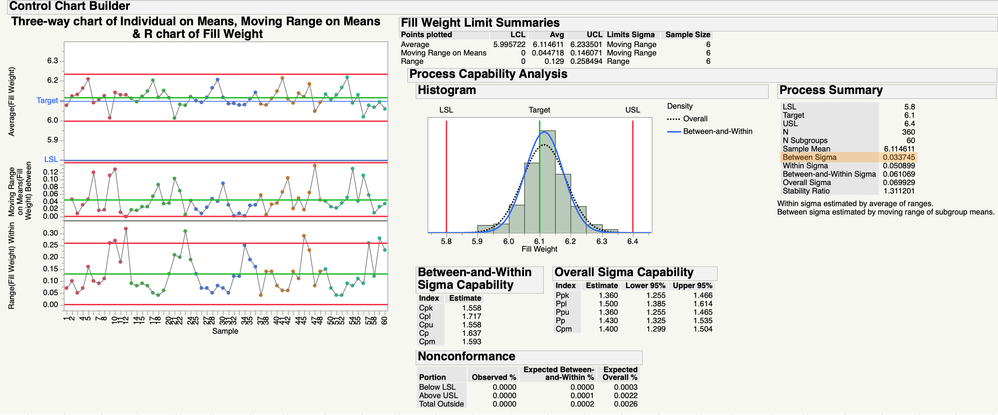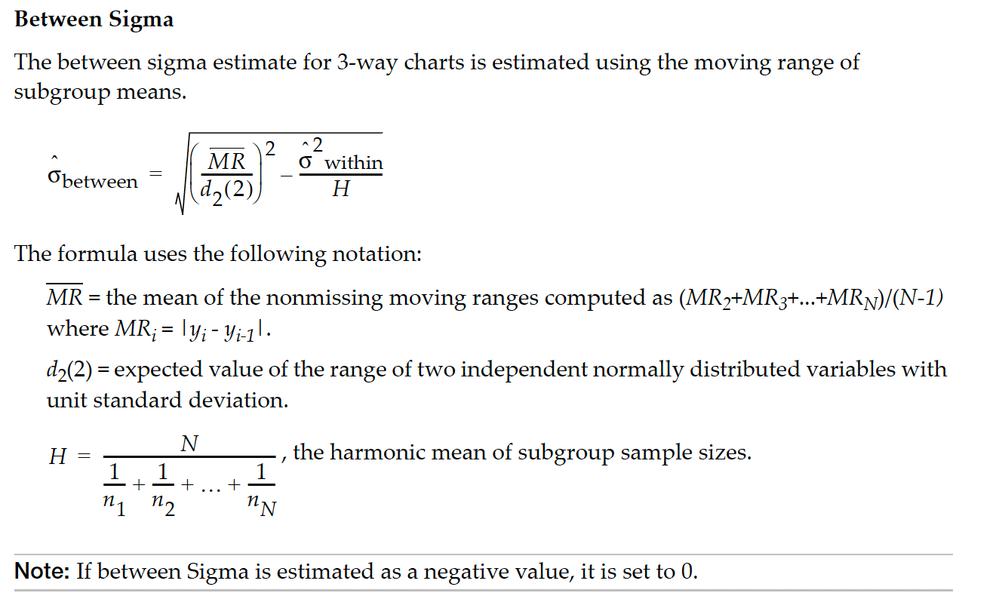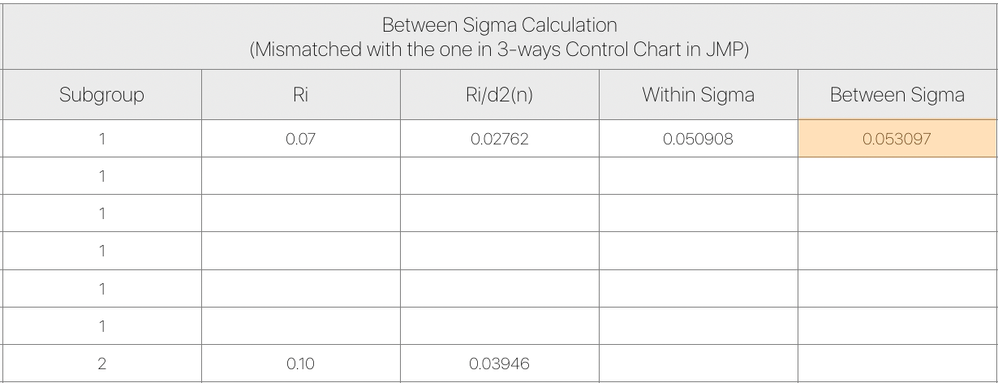Choose Language Hide Translation Bar
Highlighted

## Between Sigma Calculation Mismatch issue in 3-way Control Chart

Hello,

After carefully validation in terms of Between Sigma by using 3-Way Control Chart,
I observed there is mismatch about Between Sigma calculation in 3-Way Control Chart and the formula provided by JMP statistics document.
I used the data library example 'Vial Fill Weights.jmp' as an example.The Between Sigma in 3-Ways Control Chart is 0.033745

In JMP document, the between sigma calculation is:I put the raw data of Vial Fill Weights in Excel file and follow the formula above to calculate the Between Sigma.
But the calculation results are quite different (mismatched compared with the one in 3-Way Control Chart):I validate the Overall Sigma, Within Sigma, Sigma for Subgroups (n=6 in this example), all those calculation results fit very well.
But only found mismatch for Between Sigma calculation.
Could you help to double check what's the issue?
What's the exact formula being used in 3-Way Control Chart in JMP for Between Sigma calculation?
Thank you very much!
(Detailed calculation file attached)
1 ACCEPTED SOLUTION

Accepted Solutions
Highlighted

## Re: Between Sigma Calculation Mismatch issue in 3-way Control Chart

=(G7^2-O3^2/6)^0.5

I'm not sure where the subgroup means are calulated, I can see the subgroup ranges but of course that's something different too.

I used this formula to replicate the calculation in JMP

Sqrt( Col Mean( :Moving Range of Subgroup Means / :d2 2 ) ^ 2 - :Within Sigma ^ 2 / (60 / (60 * (1 / 6))) )

The harmonic mean is represented with this expression rather than writing the whole thing out.  (60 / (60 * (1 / 6))), its still 6 though.

JMP Systems Engineer, Pharm and BioPharm Sciences
4 REPLIES 4
Highlighted

## Re: Between Sigma Calculation Mismatch issue in 3-way Control Chart

=(G7^2-O3^2/6)^0.5

I'm not sure where the subgroup means are calulated, I can see the subgroup ranges but of course that's something different too.

I used this formula to replicate the calculation in JMP

Sqrt( Col Mean( :Moving Range of Subgroup Means / :d2 2 ) ^ 2 - :Within Sigma ^ 2 / (60 / (60 * (1 / 6))) )

The harmonic mean is represented with this expression rather than writing the whole thing out.  (60 / (60 * (1 / 6))), its still 6 though.

JMP Systems Engineer, Pharm and BioPharm Sciences
Highlighted

## Re: Between Sigma Calculation Mismatch issue in 3-way Control Chart

Thanks Byron!

I think the root cause is that I didn't use mean of moving range on means as MR for the calculation.

I used individual MR (n=2) instead which caused the Between Sigma results mismatched.

Do you know why we need mean of moving range on means? Do you know the realistic explaination behind this calculation? Appreciate it.

Highlighted

## Re: Between Sigma Calculation Mismatch issue in 3-way Control Chart

1. Control chart theory is pretty important. I like the Donald Wheeler text books for the practical approach, also Douglas Montgomery has a more mathematical and theoretical text book that is very good too.

2. If you trying to "validate" JMP with Excel, your're likely to run into some issues with Excel.

JMP's numerical accuracy is vetted extensively, and all the methods in JMP, especially in the quality tools, are from referenced sources.